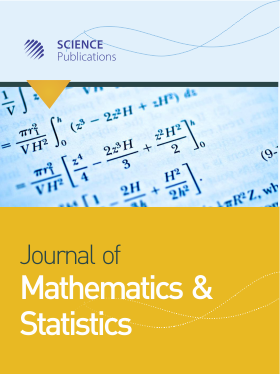Frequency: Continuous
ISSN: 1549-3644 (Print)
ISSN: 1558-6359 (Online)
Research Article Open Access

# New Family of Exact Soliton Solutions for the Nonlinear Three-Wave Interaction Equations

Sahar M. Alqaraleh1 and Adeeb G. Talafha1
• 1 ,
Journal of Mathematics and Statistics
Volume 7 No. 1, 2011, 45-50

DOI:

Submitted On: 15 August 2010 Published On: 31 January 2011

How to Cite: Alqaraleh, S. M. & Talafha, A. G. (2011). New Family of Exact Soliton Solutions for the Nonlinear Three-Wave Interaction Equations. Journal of Mathematics and Statistics, 7(1), 45-50. https://doi.org/10.3844/jmssp.2011.45.50

## Abstract

Problem statement: To obtain new exact traveling wave soliton solutions for the threewave interaction equation in a dispersive medium and a non zero phase mismatch. Approach: The tanh method is usually used to find a traveling wave analytic soliton solutions for one nonlinear wave and evolution partial differential equation. Here, we generalize this method to solve a system of nonlinear evolution partial differential equations, then we use this generalization to find new family of exact traveling wave soliton solutions for the nonlinear three-wave interaction equation. Results: We were able to generalize the tanh method and apply this generalization to the (TWI) system of (PDE’s). We derive a system of algebraic Eq. 28-32 and introduced some interested sets of solutions for this system, these sets of solutions leads us to write explicit analytic new family of soliton solutions for the three-wave interaction equation. Conclusion: The generalization of the tanh method is proved its efficiency in obtaining exact solutions for nonlinear evolution partial differential equations. This method also can be used similarly to obtain exact solutions for another interested nonlinear evolution system of partial differential equations.

• 1,072 Views